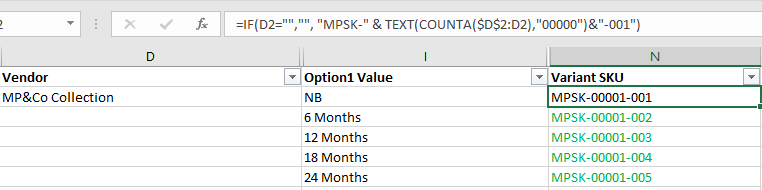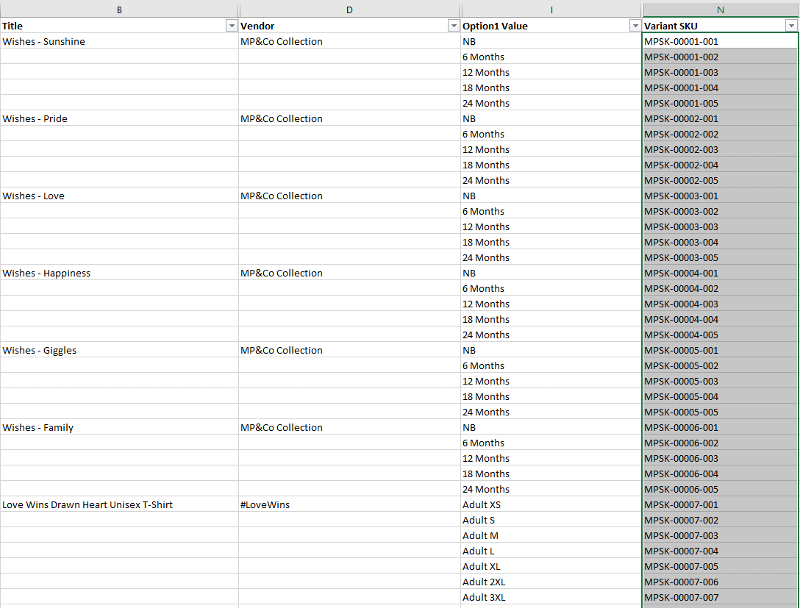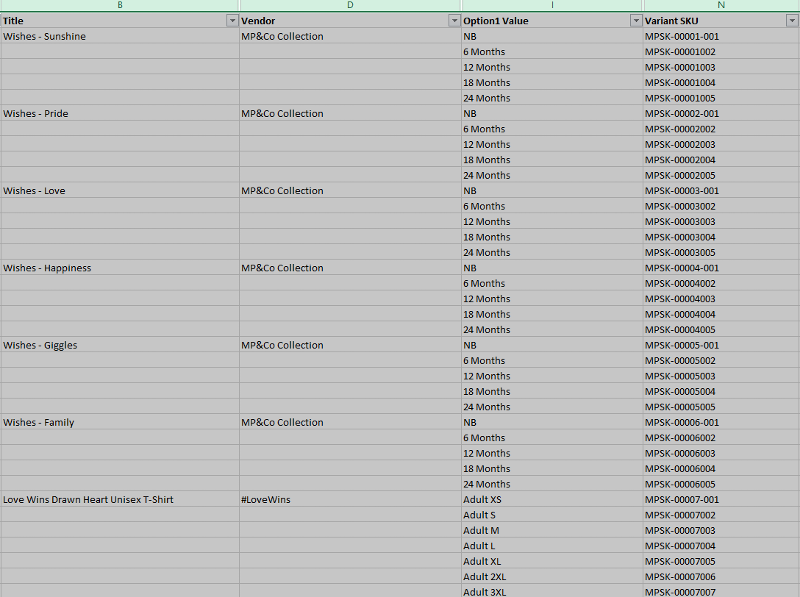# Excel Formula: How to increment-alphanumeric-column-by-1 Part 2mrrmpc used Ask the Experts™
on
This is a follow up to previous question posted:
https://www.experts-exchange.com/questions/28960037/Excel-Formula-How-to-increment-alphanumeric-column-by-1.html?anchor=a41732303#a41732303

Received a valid, correct answer to that post (thank you Ryan).  I would like to go ahead an inquire and expand on the previous question posted.

My SKU pattern is MPSK-00001-001.  The prefix of the pattern is constant "MPSK". The dashes are part of the pattern and must be retained. And finally must retain leading zeros, padding zeros.

Would like to auto increment the middle part of the pattern the "00001" portion of the pattern +1 when column D(vendor) has a value.  From a previous post I applied the formula =IF(D2="","", "MPSK-" & TEXT(COUNTA(\$D\$2:D2),"00000")&"-001") and this produced desired results to generate the main SKU base.

-ELSE-

When column D (vendor)  is blank increment the SKU suffix portion of the pattern +1.  How to nest IF, or how to accomplish the else portion of this question?

The datasheet that I have in Excel I can not add more columns.  So I need to apply the formula starting from column N, and carry it forward to however far I need to drag it down in the future as the list grows.

Screen shot items in column N(variant SKU) black color were populated with the formula of =IF(D2="","", "MPSK-" & TEXT(COUNTA(\$D\$2:D2),"00000")&"-001") , items in green are the desired additional output.  How to extend the previous code formula is the follow up question.Comment
Watch Question

Do more withEXPERT OFFICE® is a registered trademark of EXPERTS EXCHANGE®
Excel & VBA Expert
Most Valuable Expert 2018
Awarded 2015

Commented:

In N2
``````=IF(D2&I2="","",IF(D2<>"","MPSK-"&TEXT(COUNTA(\$D\$2:D2),"00000")&"-001","MPSK-"&TEXT(COUNTA(\$D\$2:D2),"00000")&TEXT(RIGHT(N1,3)+1,"000")))
``````
and copy it down.
Top Expert 2016
Commented:
Hi,

pls try

``````= "MPSK-" & TEXT(COUNTA(\$D\$2:D2),"00000")&"-"&TEXT(IF(D2="",RIGHT(N1,3)+1,1),"000")
``````
Excel & VBA Expert
Most Valuable Expert 2018
Awarded 2015
Commented:
@Rgonzo

In your previous formula, you were referring to col. G. Why? Though you tweaked it later.

Also your tweaked formula will keep populating col. N even if there is not data in col. D. ;)
Top Expert 2016

Commented:
@Neeraj
That's right but looking at the example in the first question it shouldn't be a problem
Excel & VBA Expert
Most Valuable Expert 2018
Awarded 2015

Commented:
I think user always copy such formulas down the rows in advance and like the formula cells to populate automatically once they fill the column of interest. That's the usual practice. :)
Top Expert 2016

Commented:
then use
``````=IF(D2&I2="","","MPSK-" & TEXT(COUNTA(\$D\$2:D2),"00000")&"-"&TEXT(IF(D2="",RIGHT(G1,3)+1,1),"000"))
``````

Commented:
In testing the code solutions out, thank you both for responding so quickly, both appeared to work.  The file right now has over 2K row entries that needed to be SKU'd.  The only difference I caught was the loss of the dash in suffix portion of the sku pattern when tested @Neeraj though I modified and appeared to work.

Again thank you both very much.

@Rgonzo
= "MPSK-" & TEXT(COUNTA(\$D\$2:D2),"00000")&"-"&TEXT(IF(D2="",RIGHT(N1,3)+1,1),"000")@ Neeraj
=IF(D2&I2="","",IF(D2<>"","MPSK-"&TEXT(COUNTA(\$D\$2:D2),"00000")&"-001","MPSK-"&TEXT(COUNTA(\$D\$2:D2),"00000")&TEXT(RIGHT(N1,3)+1,"000")))Excel & VBA Expert
Most Valuable Expert 2018
Awarded 2015

Commented:
Ah that means I posted the wrong formula. I corrected it but forgot to post and posted the wrong one instead.